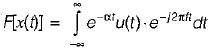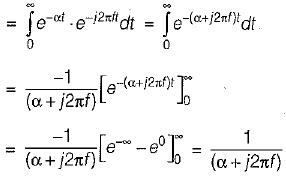Courses

# Test: Fourier Analysis of Signals, Energy & Power Signals

## 10 Questions MCQ Test Topicwise Question Bank for Electronics Engineering | Test: Fourier Analysis of Signals, Energy & Power Signals

Description
This mock test of Test: Fourier Analysis of Signals, Energy & Power Signals for Electronics and Communication Engineering (ECE) helps you for every Electronics and Communication Engineering (ECE) entrance exam. This contains 10 Multiple Choice Questions for Electronics and Communication Engineering (ECE) Test: Fourier Analysis of Signals, Energy & Power Signals (mcq) to study with solutions a complete question bank. The solved questions answers in this Test: Fourier Analysis of Signals, Energy & Power Signals quiz give you a good mix of easy questions and tough questions. Electronics and Communication Engineering (ECE) students definitely take this Test: Fourier Analysis of Signals, Energy & Power Signals exercise for a better result in the exam. You can find other Test: Fourier Analysis of Signals, Energy & Power Signals extra questions, long questions & short questions for Electronics and Communication Engineering (ECE) on EduRev as well by searching above.
QUESTION: 1

### The signals x1(t) and x2(t) are given by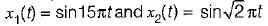If the periods of x1(t) and x2(t) be T1 and T2 respectively, then T1/T2 is equal to

Solution:

x1(t) = sin15πt is a periodic signal having period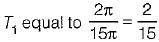x2(t) = sin√2 πt is a periodic signal having period T2 equal to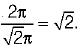∴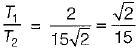QUESTION: 2

### The Fourier transform of a unit step function is

Solution: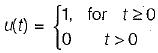∴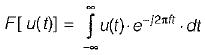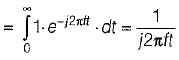QUESTION: 3

### Assertion (A): A frequency domain representation of a signal shows the frequency content and indicates the shape of the waveform or the combined amplitude of all the input components at any specific time. Reason (R): In frequency domain, a signal is represented by its frequency spectrum.

Solution:

A frequency domain representation of a signal shows the frequency content but not necessarily indicate the shape of the waveform or the combined amplitude of all the input components at any specific time. Hence, assertion is a false statement. Reason is a true statement because in frequency domain, a signal is represented by its frequency spectrum and to obtain frequency spectrum of a signal, Fourier series and Fourier transform are used.

QUESTION: 4

Which of the following option is not correct for obtaining the Fourier transform of a signal x(f)7

Solution:

For obtaining the Fourier transform of a signal x(t), it must have infinite number of discontinuities in any finite interval T.

QUESTION: 5

Match List-I (Operations on x(t)) with List-ll (X(ω)/Fourier transform) and select the correct answer using the codes given below the lists: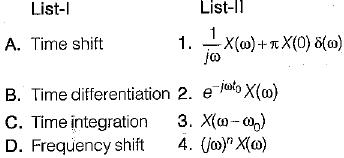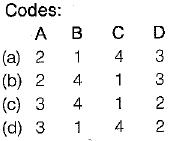Solution: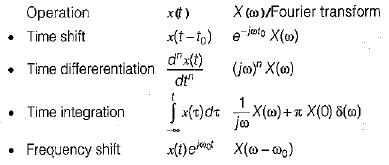QUESTION: 6

Assertion (A): The Parseval’s theorem implies superposition of the average powers.
Reason (R): The interpretation of the Parseval’s theorem is that the total average power of the signal x(t) can be found by squaring and adding the heights of the amplitude lines in the spectrum of the periodic signal x(t).

Solution:
QUESTION: 7

Fourier series analysis is a tool used to
1. analyse any periodic and non-periodic signal.
2. find how many frequency components are present in the signal.
3. find the relative phase difference between various frequency components.
4. represents a waveform in the form of sum of infinite number of exponential terms.
5. find the amplitude of various terms in the series.
Which of the statements given above are correct?

Solution:

Fourier series analysis is a tool used to
1. analyse any periodic signal only.
2. find how many frequency components are present in the signal.
3. find the relative phase difference between various frequency components.
4. represents a periodic waveform in the form of sum of infinite number of sine and cosine terms.
5. find the amplitude of various frequency components of the series.
Hence, statements 2, 3 and 5 are only correct.

QUESTION: 8

The power spectral density of a power signal x(t) is shown in figure below.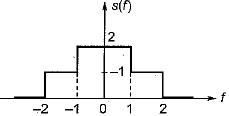The average power of the signal is

Solution:

The total average power of a signal is the area of curve under s(f).
Hence,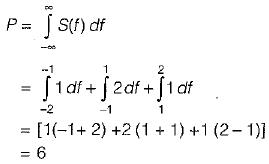QUESTION: 9

The energy of a causal exponential pulse shown in figure is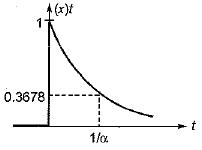Solution:

Given,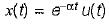∴ Normalized energy of given signal is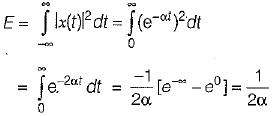QUESTION: 10

The Fourier transform of the decaying exponential pulse shown in figure is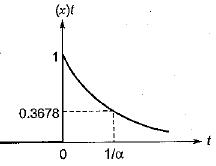Solution:

The given signal can be represented as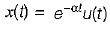(Time constant,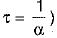∴ Fourier transform of x(t) is